• python画图-如何用python画图
千次阅读 多人点赞
2020-11-01 12:59:52python2.6后引入一个叫做海龟绘图(Turtle Graphics)的绘图工具。turtle库是python的内部库，直接使用import turtle即可。

思路：

1. 确定好需要画的图。

2. 创建一个画布，用来画你需要的图。

（1）画布大小，可以使用默认大小，也可以自定义画布大小。

（2）画布背景色bgcolor()。

（3）确定起点位置。

3. 画笔的设置。

（1）画笔的大小，颜色。

（2）画笔运行属性。

二、定义画布参数import turtle as t

t.screensize(width = None,heigh = None,bg = None)　　#以像素为单位，参数分别为画布的宽，高，背景色

t.screensize()　　#返回默认大小（400，300）

t.Screen()　　　　#也是表示默认画布大小，注意S大写

t.setup(w=0.5,h=0.75,startx=None,starty=None)　#w,h为整数是表示像素，为小数时表示占据电脑屏幕的比例

#startx，starty坐标表示矩形窗口左上角顶点的位置，默认为屏幕中心位置

三、定义画笔

1、画笔的状态

在画布上默认有一个坐标原点为画布中心的坐标轴，坐标原点上有一只面朝x轴正方向小乌龟。这里我们描述小乌龟时使用了两个词语：坐标原点(位置)，面朝x轴正方向(方向)，turtle绘图中，就是使用位置方向描述小乌龟(画笔)的状态。

2、画笔的属性

画笔(画笔的属性，颜色、画线的宽度)

（1）turtle.pensize()：设置画笔的宽度；

（2）turtle.pencolor()：没有参数传入，返回当前画笔颜色，传入参数设置画笔颜色，可以是字符串如"green"，"red"，也可以是RGB 3元组；>>> pencolor('brown')

>>> tup = (0.2, 0.8, 0.55)

>>> pencolor(tup)

>>> pencolor()

'#33cc8c'

（3）turtle.speed(speed)：设置画笔移动速度，画笔绘制的速度范围[0,10]整数，数字越大越快。

3、绘图命令

操纵海龟绘图有着许多的命令，这些命令可以划分为3种：一种为运动命令，一种为画笔控制命令，还有一种是全局控制命令。

（1）画笔运动命令：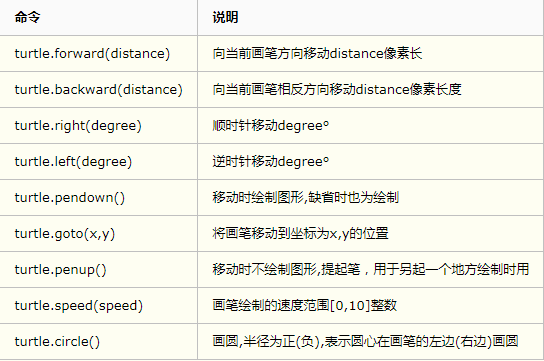（2）画笔控制命令：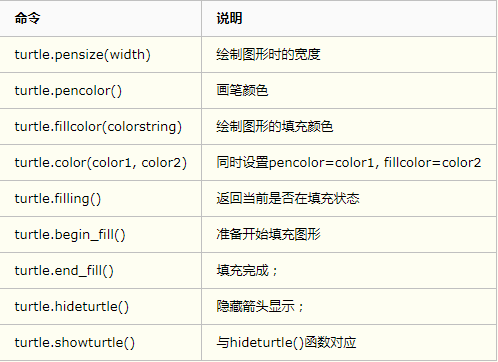（3）全局控制命令：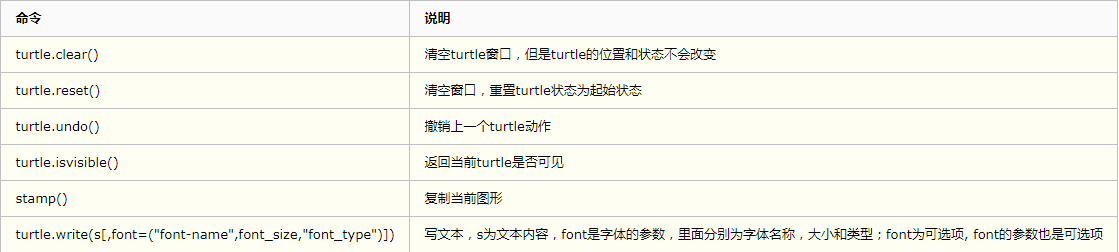描述：以给定半径画圆

参数：

extent(弧度) (optional)；

举例：circle(50) # 整圆;

circle(50,steps=3) # 三角形;

circle(120, 180) # 半圆

五、绘图举例

（1）菱形太阳花import turtle as t #turtle库是python的内部库，直接import使用即可

def draw_diamond(turt):

for i in range(1,3):

turt.forward(100) #向前走100步

更多相关内容
• 利用python读取excel表格数据并且画折线图这个基础的入门级代码，具有很好的参考学习价值，用到python的两个库这两个库要自己去下载，代码能运行
• 今天小编就为大家分享一篇python画图——实现在图上标注上具体数值的方法，具有很好的参考价值，希望对大家有所帮助。一起跟随小编过来看看吧
• 今天小编就为大家分享一篇python画图把时间作为横坐标的方法，具有很好的参考价值，希望对大家有所帮助。一起跟随小编过来看看吧
• 根据网上查到的蝴蝶计算公式，自己尝试用python turtle库制作了一个化蝶曲线生成的动画，算是对自己自学Python的奖励。
• 自己网上没事干学的小程序，希望大家学习，一起来学pythyon
• 在介绍Python画图模板之前，先买个和大家卖个官司，首先介绍一下Python画图常用库matplotlib的参数，如果只介绍模板，如果你想改一些参数，首先要知道各个参数的含义，那么就可以去这个网址查找了： matplotlib官方...
• python画图表，这里的图表为散点图和饼状图，可以被广泛地用于工作和学习的需要中，并且代码简洁易懂。
• python中的turtle库，for循环画出五环，五环颜色不同，熟悉一下python的turtle库
• 对很多的代码也是非常陌生，高中忙于学习的我们甚至可以说是对编程是一无所知，进入大学进入到这个专业才开始接触很多电脑相关的东西才开始接触编程，下面我就教大家如何利用编程语言画图，以Python语言为例，我们...
• 可以顺畅的再Python中调取Excel文件，并用值进行画图，好用
• pythonpythonpython 画图介绍 本文以实用为第一目标，保证读者在看完此文之后可以迅速上手 pythonpythonpython 画图，掌握所有画图的基本技巧。 库加载 我们使用 matplotlib 来进行 pythonpythonpython 画图，具体...

### p y t h o n python 画图介绍 嘿！彩蛋！感觉有帮助就三连呗！

本文以实用为第一目标，保证读者在看完此文之后可以迅速上手 p y t h o n python 画图，掌握所有画图的基本技巧。（收藏的同时点个赞呗ヽ(•̀ω•́ )ゝ）

如果你对这篇文章可感兴趣，可以点击「【访客必读 - 指引页】一文囊括主页内所有高质量博客」，查看完整博客分类与对应链接。

相关文章：

库加载

我们使用 matplotlib 来进行 p y t h o n python 画图，具体的库加载以及初始设置代码如下所示。

import numpy as np	# 加载数学库用于函数描述
import matplotlib
import matplotlib.pyplot as plt
from matplotlib import style

matplotlib.rcParams['text.usetex'] = True  # 开启Latex风格
plt.figure(figsize=(10, 10), dpi=70)  # 设置图像大小
# style.use('ggplot')  # 加载'ggplot'风格
# f, ax = plt.subplots(1, 3)  # 设置子图


每一段代码都有注释，可以根据注释把握每行代码的内容。看不懂也没有关系，先把代码加上，继续往下看即可。

p y t h o n python 画图的原理

其实原理非常简单，就是给出一个横坐标的 l i s t list ，再给出一个对应的纵坐标 l i s t list ，然后设置点大小、点外框大小、线大小、点颜色、线颜色、点形状、线形状、标题、标签即可。设置部分的内容看起来很多，但其实也可以不用设置，不设置就代表使用默认的大小、形状以及颜色。

除此之外，画图最主要的分类就是画点还是将点连线，如果使用 scatter 函数，则将散点画在图中；如果使用 plot 函数，则会将散点连线，在下文中，读者将会更深刻地感受到这一点。

图像加载

# plt.tight_layout() # 当有多个子图时，可以使用该语句保证各子图标题不会重叠
# plt.savefig('myplot1.pdf', dpi=700) # dpi 表示以高分辨率保存一个图片文件，pdf为文件格式，输出位图文件
plt.show() # 渲染图片


上述的代码中主要就是 plt.show() 语句，其余语句都是一些其余设置，看注释即可明白。

画图分类

python 中画图主要分为下述几类。

• 画单点
• 画散点
• 画散点连线
• 画函数

主要涉及到的一些 操作 分为下述几类。

• 子图
• 设置横纵坐标范围
• 图像标题
• 图像中画图类型的标签

### 画单点

画单点比较简单，给出下述代码以及返回结果即可掌握。（需要加入最开头给出的初始设置内容）

def main():
X = 1
Y = 100
plt.scatter(X, Y, s=50)	// s为点大小
plt.show()上图是加载了 style.use('ggplot') 该风格后的效果，如果去掉该行，输出将如下所示。读者可以根据自己的喜好选择该风格，也可以去搜索其他的风格，下文接下来的示例将均使用 'ggplot' 风格进行展示。

### 画散点

知道了画单点的方法之后，画散点就变得非常简单，因为我们只需要将单点中传入的单变量改成一个 l i s t list 即可，具体代码和效果如下所示。

def main():
X, Y = [], []
for i in range(1, 10):
X.append(i)
Y.append(np.sin(i))
plt.scatter(X, Y, s=50)
plt.show()### 散点连线

只有散点，通常会使得图像变得不够直观，因此我们使用 plot 函数将所有散点连在一起，具体操作如下所示。

def main():
X, Y = [], []
for i in range(1, 10):
X.append(i)
Y.append(np.sin(i))
plt.plot(X, Y, s=50)
plt.show()我们也可以对 plot 函数进行设置，即可输出如下所示图形。

def main():
X, Y = [], []
for i in range(1, 10):
X.append(i)
Y.append(np.sin(i))
plt.plot(X, Y, '-p', color='grey',
marker = 'o',
markersize=8, linewidth=2,
markerfacecolor='red',
markeredgecolor='grey',
markeredgewidth=2)
plt.show()可以看到我们对于点大小、颜色、点外框颜色、大小以及线颜色、线宽均进行了设置，读者可以自行选择设置选项进行尝试。

### 画函数

尽管散点连线了，但是由于散点的密度不够大，因此我们可以进一步的使用
只有散点，通常会使得图像变得不够直观，因此我们使用 plot 函数将所有散点连在一起，具体操作如下所示。

def main():
X = np.linspace(1, 10, 100)	# 将[1,10]区间均分为100个点，得到100个横坐标
Y = np.sin(X) # 求出100个点的纵坐标
plt.plot(X, Y, color="red", linewidth=1.0, linestyle="-") # 将100个散点连在一起
plt.show()线条形状、颜色、标签

在画函数中我们可以设置线条形状 linestyle 、线条宽度 linewidth 以及线条颜色 color。我们接下来介绍常见的几种线条形状以及如何给线条加上标签，并给出具体的代码供读者参考。

def main():
X = np.linspace(1, 10, 100)
Y1 = np.sin(X)
Y2 = np.cos(X)
Y3 = -X
Y4 = X
plt.plot(X, Y1, color="lightcoral", linewidth=3.0, linestyle="-", label="-")
plt.plot(X, Y2, color="burlywood", linewidth=3.0, linestyle="--", label="--")
plt.plot(X, Y3, color="mediumturquoise", linewidth=3.0, linestyle="-.", label="-.")
plt.plot(X, Y4, color="mediumpurple", linewidth=3.0, linestyle=":", label=":")
plt.legend(loc="best") # 把标签加载到图中哪个位置
plt.show()


常见的 linestyle 还有 '-', '--', '-.', ':', 'solid', 'dashed', 'dashdot', 'dotted'，读者可以自行尝试。
常见的 legendloc 位置还有 best, upper right, upper left, lower left, lower right, right, center left, center right, lower center, upper center, center，其中 best 表示将标签加载到 python 认为最佳的位置。支持 Latex 的标签

由于是函数作图，因此我们通常会将标签设置为线条所代表的函数值，因此我们需要介绍支持 Latex 的标签。我们将 label 的数值设置为 r'$x_n$' 即可支持 L a t e x Latex ，如下图所示。

def main():
X = np.linspace(0, 1, 100)
Y1 = np.sqrt(X)
Y2 = X
Y3 = X * X
Y4 = X * X * X
plt.plot(X, Y1, color="lightcoral", linewidth=3.0, linestyle="-", label=r"$y_1=\sqrt{x}$")
plt.plot(X, Y2, color="burlywood", linewidth=3.0, linestyle="--", label=r"$y_2=x$")
plt.plot(X, Y3, color="mediumturquoise", linewidth=3.0, linestyle="-.", label=r"$y_3=x^2$")
plt.plot(X, Y4, color="mediumpurple", linewidth=3.0, linestyle=":", label=r"$y_4=x^3$")
plt.legend(loc="best")
plt.show()设置横纵坐标标号以及图像标题

最后我们需要介绍如何给该图像设置横纵坐标、范围以及标题。我们首先不设置范围，则具体代码以及输出图形如下。

def main():
X = np.linspace(0, 1, 100)
Y1 = np.sqrt(X)
Y2 = X
Y3 = X * X
Y4 = X * X * X
plt.plot(X, Y1, color="lightcoral", linewidth=3.0, linestyle="-", label=r"$y_1=\sqrt{x}$")
plt.plot(X, Y2, color="burlywood", linewidth=3.0, linestyle="--", label=r"$y_2=x$")
plt.plot(X, Y3, color="mediumturquoise", linewidth=3.0, linestyle="-.", label=r"$y_3=x^2$")
plt.plot(X, Y4, color="mediumpurple", linewidth=3.0, linestyle=":", label=r"$y_4=x^3$")
plt.legend(loc="best")

# x、y坐标以及标题
plt.xlabel('x', fontsize=18)
plt.ylabel('y', fontsize=18)
plt.title(r'$f(x)=\sqrt{x},x,x^2,x^3$', fontsize=18)
plt.show()设置横纵坐标的范围

有的时候我们会觉得系统默认的横纵坐标范围不合适，因此我们需要自行设置横纵坐标，具体代码以及结果如下所示。

def main():
X = np.linspace(0, 2, 100)
Y1 = np.sqrt(X)
Y2 = X
Y3 = X * X
Y4 = X * X * X
plt.plot(X, Y1, color="lightcoral", linewidth=3.0, linestyle="-", label=r"$y_1=\sqrt{x}$")
plt.plot(X, Y2, color="burlywood", linewidth=3.0, linestyle="--", label=r"$y_2=x$")
plt.plot(X, Y3, color="mediumturquoise", linewidth=3.0, linestyle="-.", label=r"$y_3=x^2$")
plt.plot(X, Y4, color="mediumpurple", linewidth=3.0, linestyle=":", label=r"$y_4=x^3$")
plt.legend(loc="best")

# x、y坐标以及标题
plt.xlabel('x', fontsize=18)
plt.ylabel('y', fontsize=18)
plt.title(r'$f(x)=\sqrt{x},x,x^2,x^3$', fontsize=18)

# 设置坐标范围
plt.ylim(-1, 4)
plt.xlim(-1, 4)
plt.show()给函数中的一个点标号

当函数比较复杂的时候，我们往往需要对关键点进行标号，通常使用的方式是加箭头或者不加，我们先给出加箭头的代码以及结果。

def main():
X = np.linspace(0, 1, 100)
Y1 = np.sqrt(X)
Y2 = X
Y3 = X * X
Y4 = X * X * X
plt.plot(X, Y1, color="lightcoral", linewidth=3.0, linestyle="-", label=r"$y_1=\sqrt{x}$")
plt.plot(X, Y2, color="burlywood", linewidth=3.0, linestyle="--", label=r"$y_2=x$")
plt.plot(X, Y3, color="mediumturquoise", linewidth=3.0, linestyle="-.", label=r"$y_3=x^2$")
plt.plot(X, Y4, color="mediumpurple", linewidth=3.0, linestyle=":", label=r"$y_4=x^3$")
plt.legend(loc="best")

# x、y坐标以及标题
plt.xlabel('x', fontsize=18)
plt.ylabel('y', fontsize=18)
plt.title(r'$f(x)=\sqrt{x},x,x^2,x^3$', fontsize=18)

# 关键点标号
plt.scatter(1, 1, s=100)
plt.ylim(-1, 2)
plt.annotate('End Point', fontsize=20,
xy=(1, 1), xycoords='data',
xytext=(0.8, 0.95), textcoords='axes fraction',
arrowprops=dict(facecolor='black', shrink=0.1),
horizontalalignment='right', verticalalignment='top')
plt.show()


其中 xy(1,1) 表示关键点的位置，xycoords='data' 表示关键点坐标所采用的坐标方式， shrink 参数表示箭头距离关键点的距离，horizontalalignment='right', verticalalignment='top' 均表示注释的位置。也可以使用不加箭头的方式，即将上述 annotate 换成下述代码即可。

	# 关键点标号
plt.scatter(1, 1, s=100)
plt.ylim(-0.1, 1.3)
plt.xlim(-0.1, 1.3)
plt.annotate("End Point",
xy=(1.05, 1.05),
fontsize=20,
xycoords="data")色卡

当绘制多个函数时，会发现颜色的设计非常难把握，因此下图给出一张色卡供颜色选择。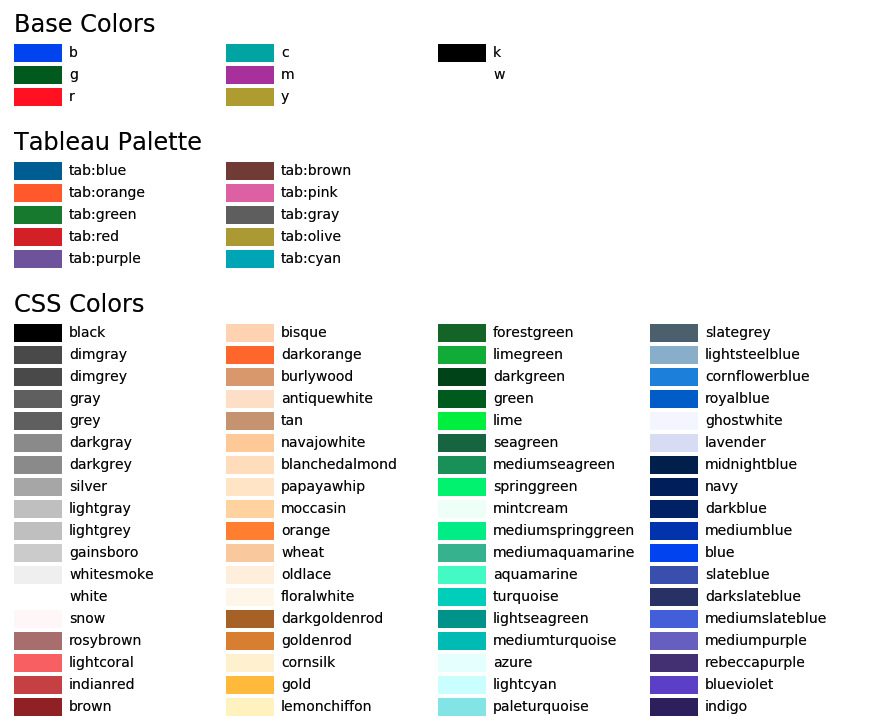### 子图

最后是如何用 python 画子图，其实画子图就是将上述的 p l t plt 换成 a x ax ，具体操作的思路与之前的操作并没有太大的区别，接下来给出一个包含横纵坐标、函数坐标、标题的完整子图代码，读者可以从代码中获取相应操作的代码。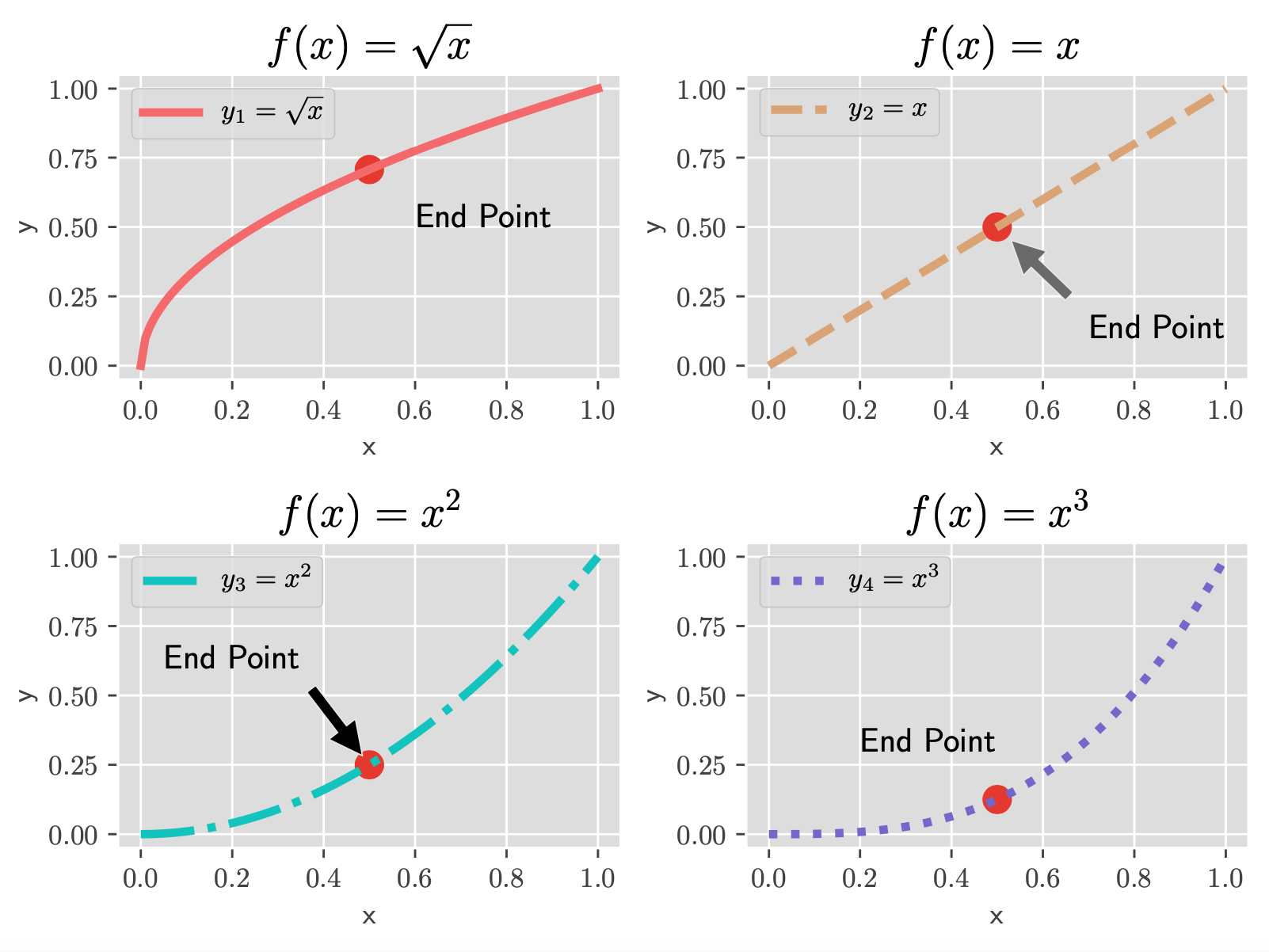import numpy as np
import matplotlib
import matplotlib.pyplot as plt
from matplotlib import style

style.use('ggplot')  # 加载'ggplot'风格
matplotlib.rcParams['text.usetex'] = True  # 开启Latex风格
plt.figure(figsize=(10, 10), dpi=70)  # 设置图像大小
f, ax = plt.subplots(2, 2)  # 设置子图

def func1():
X = np.linspace(0, 1, 100)
Y1 = np.sqrt(X)
# ax的设置
ax.plot(X, Y1, color="lightcoral", linewidth=3.0, linestyle="-", label=r"$y_1=\sqrt{x}$")
ax.set_xlabel('x', fontsize=10)
ax.set_ylabel('y', fontsize=10)
ax.set_title(r'$f(x)=\sqrt{x}$', fontsize=16)
ax.legend(loc="best")

# ax关键点
ax.scatter(0.5, np.sqrt(0.5), s=100)
ax.annotate("End Point",
xy=(0.6, 0.5),
fontsize=12,
xycoords="data")

def func2():
X = np.linspace(0, 1, 100)
Y2 = X
# ax的设置
ax.plot(X, Y2, color="burlywood", linewidth=3.0, linestyle="--", label=r"$y_2=x$")
ax.set_xlabel('x', fontsize=10)
ax.set_ylabel('y', fontsize=10)
ax.set_title(r'$f(x)=x$', fontsize=16)
ax.legend(loc="best")

# ax关键点
ax.scatter(0.5, 0.5, s=100)
ax.annotate("End Point",
fontsize=12,
xytext=(0.7, 0.1),
xy=(0.5, 0.5),
xycoords="data",
arrowprops=dict(facecolor='gray', shrink=0.15))

def func3():
X = np.linspace(0, 1, 100)
Y3 = X * X
# ax的设置
ax.plot(X, Y3, color="mediumturquoise", linewidth=3.0, linestyle="-.", label=r"$y_3=x^2$")
ax.set_xlabel('x', fontsize=10)
ax.set_ylabel('y', fontsize=10)
ax.set_title(r'$f(x)=x^2$', fontsize=16)
ax.legend(loc="best")

# ax关键点
ax.scatter(0.5, 0.5 * 0.5, s=100)
ax.annotate("End Point",
fontsize=12,
xytext=(0.05, 0.6),
xy=(0.5, 0.5 * 0.5),
xycoords="data",
arrowprops=dict(facecolor='black', shrink=0.1))

def func4():
X = np.linspace(0, 1, 100)
Y4 = X * X * X
# ax的设置
ax.plot(X, Y4, color="mediumpurple", linewidth=3.0, linestyle=":", label=r"$y_4=x^3$")
ax.set_xlabel('x', fontsize=10)
ax.set_ylabel('y', fontsize=10)
ax.set_title(r'$f(x)=x^3$', fontsize=16)
ax.legend(loc="best")

# ax关键点
ax.scatter(0.5, 0.5 * 0.5 * 0.5, s=100)
ax.annotate("End Point",
xy=(0.2, 0.3),
fontsize=12,
xycoords="data")

def main():
func1()
func2()
func3()
func4()

plt.tight_layout()  # 当有多个子图时，可以使用该语句保证各子图标题不会重叠
plt.savefig('myplot1.pdf', dpi=700)  # dpi 表示以高分辨率保存一个图片文件，pdf为文件格式，输出位图文件
plt.show()

if __name__ == "__main__":
main()



另外，也可以使用 plt.sca() 来定位子图，如下述例子：

f, ax = plt.subplots(2, 3, figsize=(15, 10)) # 声明 2 行 3 列的子图
f.suptitle("设置总标题", fontsize=18)

plt.sca(ax[0, 1]) # 定位到第 1 行第 2 列的子图
# 接下来直接使用 plt 画图即可


### 后记

上述所涉及的内容即为 p y t h o n python 应用 matplotlib 画图的基本操作了，如果想要进一步的深化画图能力，推荐根据需求在 matplotlib 官方文档 中进行搜索。祝大家在 python 画图的路上更进一步！💪💪💪

展开全文matplotlib
• Python画图，自己整理的参考代码，适合于科研作图，多y轴作图，图形格式通用设置
• 今天做的是用python实现画图，画出你想要的图案，只要你的想象力足够丰富，以及能够合理的运用这个turtle模块，基本可以画出你想要的东西出来。 而且如果真要看turtle的画笔的效果的话，用python自带的idea来编写会...广告关闭

腾讯云11.11云上盛惠 ，精选热门产品助力上云，云服务器首年88元起，买的越多返的越多，最高返5000元！

今天做的是用python实现画图，画出你想要的图案，只要你的想象力足够丰富，以及能够合理的运用这个turtle模块，基本可以画出你想要的东西出来。 而且如果真要看turtle的画笔的效果的话，用python自带的idea来编写会比较方便，只要输入了画笔的高度就会出现画笔的窗口了，而且你每添加他会跟着变化 ? 一般的话，你要想...

这篇笔记依然是在做《python语言程序设计》第5章循环的习题。 其中有两类问题需要记录下来。 第一是如何画围棋棋盘。 围棋棋盘共有19纵19横。 其中，位于（0，0）的星位叫天元，其余8个星位坐标分别是：（-6，6），（0，6），（6，6），（-6，0），（6，0），（-6，-6），（0，-6），（6，-6）。 这里面涉及到绘制等...for letter in python: if letter == h:break print(letter)上面一段代码的运行结果如下: h 在python中，for... else结构的作用，else后面接的执行语句在...2018年6月28日笔记上午上课前分享高等数学求解及作图软件：mathmatica5. 循环嵌套? 循环嵌套1.png-38.7kb? 循环嵌套2.png-50.2kb? 循环嵌套3.png-46.8kb?...

这些是你需要告诉计算机的，只要你把这些解决好了，你会发现计算机很听话，你想让它干啥就干啥（前提是它具备这样的硬性条件啊）。 今天我们的任务就是让计算机在屏幕上输出game over这样的一个字符串。? ----运行python程序的两种方法： ? 1、交互式 打开交互命令程序，启动python解释器，弹出一个python shell窗口...

做完后，我又想到，python 既然可以轻易的完成任何的数学计算，何不用 python 开发一个小程序专门用以计算呢？ 今天就让我们走进计算器的世界，用 python 来编写一个可以用的计算器。 废话不多说，先来看看整体效果编写思路用户可以通过鼠标或键盘来输入或删除数字或符号，按下等号便可计算。 本教程借助 python 的 ...

（错）3、python 3.x和python 2.x唯一的区别就是：print在python 2.x中是输出语句，而在python 3.x中是输出函数。 （错）4、在windows平台上编写的python程序无法在unix平台运行。 （错）5、不可以在同一台计算机上安装多个python版本。 （错）6、已知 x = 3，那么赋值语句 x = abcedfg 是无法正常执行的。 （错）7...

该笔记源自尚学堂,非原创python 单词是“大蟒蛇”的意思。 但是龟叔不是喜欢蟒蛇才起这个名字，而是正在追剧：英国电视喜剧片《蒙提·派森的飞行马戏团》(monty python and the flying circus)。 使用 www.python.org 提供的 interactive shell 入门 python·特点可读性强可读性远比听上去重要的多得多。 一个程序会...introduction-to-programming-using-python-january-iap-2008http:my.oschina.nettaogangblog286955python画图%matplotlib inlinefrommpl_toolkits.mplot...函数操作函数是重用的程序段。 它们允许你给一块语句一个名称，然后你可以在你的程序的任何地方使用这个名称任意多次地运行这个语句块。 这被称为 调用 ...

在阅读本文前，请确保你已经或可能满足以下条件：电脑中已经安装配置好python3环境了解python3的基础语法，比如导入模块，基础语句，面向对象学习gui编程指南：在这个gui领域qt横行的年代，依旧有学习tkinter的价值。 要学习gui编程，你大概会经历这样一条路径：认识tkinter模块，写一个简单gui程序认识各种控件...与此同时，python的gui编程最常用的库就是tkinter，它包含了很多python交互界面编程所需要的函数。 最近就有一个客户需求在gui界面实现卡通人物的点击动作，那么今天我就来和大家分享一个在tkinter库中使用画布绘制会动的哆啦a梦的一个骚操作。 tkinter库画图基本思路在使用python的tkinter库进行界面绘图时...www.lfd.uci.edu~gohlkepythonlibs#wordcloud使用cd命令进入whl文件的路径运行这条命令： python -m pip install #导入python画图的库，词云生成库和jieba...#定义主程序接口if __name__ == __main__: url = http:www.jianshu.com js = crawljs() content = js.getarticle(url) js.save(content)2. 制作词云图工具...

燃爆，100个python实战小项目汇总！ 每一篇文章都是一个知识点，一个小小的代码实践，总有一篇是你想要的！ 实用工具 |一款丰富强大的python绘图工具python受欢迎的主要原因之一就是它丰富的工具包，画图是我们在开发过程中非常常见的一种场景，因此，画图工具自然不胜枚举。 其中，比较知名的就是matplotlib，它可以...

这样的话python在您的windows平台上的任何一个目录下都能运行； 六、python的第一个程序“hello world”print(hello world!)hello world! 【各种语言的...jqueryeasyuiangulajs ajax异步加载 highchart画图 bootstrap4、算法、设计模式 冒泡、二叉树、哈希、拆半等常见的算法学习工厂模式、单例模式、享元模式...还记得那是一个月黑风高的晚上，一位女同事让我给他讲解数据分析结果的时候，我默默的用python画了下面这张图。 依稀记得女同事看了之后，立刻转身离去，并留下了一句”哼，臭屌丝“，留下懵逼的我凌乱在风中。凌乱的我对这张图左看、右看、上看、下看，终于明白在不懂技术的妹子眼中，这种正经的图表简直就是屌丝的...

cdf图 stackoverflow上，针对该问题有多个回答，见–how to plot empirical cdf in matplotlib in python?。 其中一种方法是使用statsmodels包提供的ecdf函数–statsmodels.distributions。 示例程序如下：import numpy as npimport statsmodels.api as sm # recommended import accordingto the docsimport matplot...它的文档相当完备，并且gallery页面中有上百幅缩略图，打开之后都有源程序。 因此如果你需要绘制某种类型的图，只需要在这个页面中浏览复制粘贴一下，基本上都能搞定。 在linux下比较著名的数据图工具还有gnuplot，这个是免费的，python有一个包可以调用gnuplot，但是语法比较不习惯，而且画图质量不高。 而 matplot...

作者：令狐虫转自：迷途小书童测试环境： python版本 3. 7.0 操作系统window 7 64位 编辑器pycharm； ----1 关于安装问1：python画图用到哪些库？ 答：python常用的绘图库有：matplotlib，是最经典的python可视化绘图库。 matplotlib就是matlab+plot+library，即模仿matlab的绘图库，其绘图风格与matlab类似...

另外一个anaconda prompt是一个命令操作终端，可以利用conda命令安装你想要的包。 例如你想要安装画图包matplotlib，就可以输入 conda install matplotlib。 一切就绪后，我们打开vscode，新建一个文件，输入print ("hello world’)，然后右键选择“在终端运行python文件”，你就可以完成你的第一个python程序啦。?...高级一点的示例现在来创建一个更好的程序。 这次的程序要完成的任务是根据选择的模式在拖动鼠标时绘制矩形或者是圆圈（就像画图程序中一样）。 所以回调...使用matplotlibmatplotib 是 python 的一个绘图库，里头有各种各样的绘图方法。 # -*- coding:utf-8 -*- import numpy as np import cv2 from matplotlib ...

展开全文• ## python画图函数

千次阅读 2020-11-30 04:16:50
广告关闭腾讯云11.11云上盛惠 ，精选热门产品助力上云，云服务器首年88元起，买的越多返的越多，最高返... 在python自带的idea下到没有这种现象发生，而且还能边写代码边画图。 turtle包本身只是一个绘图库，但是配...广告关闭

腾讯云11.11云上盛惠 ，精选热门产品助力上云，云服务器首年88元起，买的越多返的越多，最高返5000元！

在pycharm的idea下，如果绘图完成后，记得调用done()函数，让窗口进入消息循环，等待被关闭。 否则，由于python进程会立刻结束，将导致窗口被立刻关闭。 在python自带的idea下到没有这种现象发生，而且还能边写代码边画图。 turtle包本身只是一个绘图库，但是配合python代码，就可以绘制各种复杂的图形。 需要注意的...

python绘图的包大家应该不会陌生，但是，对图的常规设置不一定会知道（其实自己也是才知道的），比如：坐标轴的字体大小、颜色设置； 标题的字体颜色大小设置； 线的粗细、颜色； 图片风格的设置等。 了解这些常规设置必定会让图片更加美观。 下面就具体来说说matplotlib中有哪些常规设置。 我主要总结了这几个函数...

function 的参数传递 python的变量是一个对象的引用，变量与变量之间的赋值是对同一个对象的引用，当变量重新赋值对象的时候，是将变量指向一个新的对象。 ...准备转入j2se,j2ee，这是我的主攻。 还有科学技术，作图，spider等，怎么学习，有人，给一个意见没有，感觉这里面函数参数，太多。 手上没有好的书籍...

#在当前axes上绘图plt.sca(axes2)#plt.hist(...)# 在axes2上绘图plt.show()可以看出，面向过程就是通过plt设置当前的axes，然后，用plt在当前axes上画图axesaxes对象都有拥有几个函数:axes.xlabel(...) #x轴 labelaxes.ylabel(...) #y轴 labelaxes.title(...)#axes的titleaxes.xlim(start, end)axes.ylim(start, end)...

最新再看python3，发现了一个“海龟”画图模块，就上手用了一下，主要用到一些简单的函数和列表适合初学者浏览。 windows下写的，可以使用pyinstall生成exe文件。 #copyright leoyuan 2017#mail:centos@126.com__author__ = leoyuanimport turtle as timport timeimportrandomimport sys def screenint(): t.title...

相关函数介绍1. point该数据结构表示了由其图像坐标 和 指定的2d点。 可定义为：point pt; pt.x = 10; pt.y = 8; 或者point pt = point(10, 8); 2. scalar表示了具有4个元素的数组。 次类型在opencv中被大量用于传递像素值。 本节中，我们将进一步用它来表示rgb颜色值（三个参数）。 如果用不到第四个参数，则无需...而当执行一个函数时，内存中会临时开辟一个空间，临时存放函数中的变量与值的关系，这个叫做临时名称空间，或者局部名称空间。 其实python还有一个空间叫做内置名称空间：内置名称空间存放的就是一些内置函数等拿来即用的特殊的变量：input，print，list等等，所以，我们通过画图捋一下：? 那么这就是python中经常...

对于numpy的函数，pands等，不是很熟，我来copy一下code，敲击一下，找找感觉。 默认的导入包 import numpy as np import matplotlib.pyplot as plt defprint_line_draw(): 画直线 return:x=np.arange(0,9,1) y=x+8 plt.plot(x,y,color=red,linestyle=--,marker=*,label=y=x+8) plt.savefig(1.png,dpi=60) plt.legend...

本文由腾讯云+社区自动同步，原文地址 https:stackoverflow.clubpython-basic-api 1 简介matplotlib是python中一个非常好用的画图库，倾向于使用数据画图，设计思路与matlab中的plot相同。 1.1 画图与保存 1. 1.1 无显示器画图 ssh远程操作 出现runtimeerror: invalid display variable 添加如下代码 plt.switch_back...

使用python的turtle（海龟）模块画图第一步：让python引入turtle模块，引入模块就是告诉python你想要用它。 import turtle第二步：创建画布。 调用turtle中的pen函数。 t = turtle.pen()? 第三步：移动海龟。 t.forward(50)forward的中文意思是“向前地；促进”。 所以这行代码的意思是海龟向前移动50个像素：? t...

一、前言以前大学时候，学excel看到n多大神利用excel画图，觉得很不可思议。 今个学了一个来月python,膨胀了就想用excel画图。 当然，其实用画图这个词不甚严谨，实际上是利用opencv遍历每一个像素的rgb值，再将其转化为16进制，最后调用openpyxl进行填充即可。 1.1、实现效果效果如下图 ? 1.2、需要用到的库的安装...

qp.setpen(qt.red) size = self.size() for i in range(1000):# 两个周期的正弦函数图像 x = 100 * (-1 + 2.0 * i 1000) + size.width() 2.0 y= -50 * math.sin((x - size.width() 2.0) * math.pi 50) + size.height() 2.0qp.drawpoint(x, y)if name == main:app = qapplication(sys.argv) demo = drawing() demo...

绝大多数语言内存底层都是高度相似的，这样大家掌握了这些内容也便于以后学习其他语言。 函数简介函数的基本概念一个程序由一个个任务组成； 函数就是代表一个任务或者一个功能。 函数是代码复用的通用机制。 python 函数的分类python 中函数分为如下几类：内置函数我们前面使用的 str()、list()、len()等这些都是...

用python的matplotlib画图时，往往需要加图例说明。 如果不设置任何参数，默认是加到图像的内侧的最佳位置。 import matplotlib.pyplot as pltimport numpy as np x = np.arange(10) fig =plt.figure()ax = plt.subplot(111) for i in xrange(5):ax.plot(x, i * x, label=$y = %ix$ % i) plt.legend()plt.show()这样...

如何用python画图——带你入门matplotlib如何用python画图--matplotlib实例与补充但是matplotlib默认设置绘图效果不是非常美观，而且matpltolib在统计绘图方面也不是很给力。 而seaborn则很好的解决了这个问题。 seaborn基于matplotlib，提供了一些更美观的配置选项，而且封装了很多统计绘图函数。 所以如果你在做...introduction-to-programming-using-python-january-iap-2008http:my.oschina.nettaogangblog286955python画图%matplotlib inlinefrommpl_toolkits.mplot...函数操作函数是重用的程序段。 它们允许你给一块语句一个名称，然后你可以在你的程序的任何地方使用这个名称任意多次地运行这个语句块。 这被称为 调用 ...最近遇到个任务需要画一些坐标图，我就在想，用了这么长时间的 python 了，能不能用 python 画图呢？ 学习一门语言不能只为了学习语言而学习，要做到学以致用。 google 了一下，果然有新的发现，python 中 matplotlib 库是专门用来画图的，操作了一番后，发现用 python 画图真的爽，人生苦短，快用 python！...

说明：有部分是网上查到的案例，觉得很实用，就把它搬过来了。 ---- dataframe的列名concat拼接merge两个dataframe拼接计算nan的个数排序删除重复记录使用pandas画图中文显示问题双坐标轴的图enumerate函数时间处理时间转换为周几周月画图一个框中框中画多个图多个子图1.dataframe的列名## 方法一：全部修改df...

展开全文• 主要介绍了Python画图的方法,结合实例形式分析了Python基本的线性图、饼状图等绘制技巧,具有一定参考借鉴价值,需要的朋友可以参考下
• 主要为大家详细介绍了python实现画图工具，文中示例代码介绍的非常详细，具有一定的参考价值，感兴趣的小伙伴们可以参考一下
• 折线图plt.figure(figsize=(40, 40)) # 确定图像画布的大小plt.subplot(211) # 将画布分为两行一列plt.xlabel('Number of sample', fontsize=40) # x轴的labelplt.ylabel('Char...
•可视化
• python如何画图？这里给大家介绍两款python绘图的库：turtle和Matplotlib。相关推荐：《python视频》1 安装turtlePython2安装命令：pip install turtulePython3安装命令：pip3 install turtle2 基础概念2.1 画布...
• python如何画图？这里给大家介绍两款python绘图的库：turtle和Matplotlib。1 安装turtlePython2安装命令：pip install turtulePython3安装命令：pip3 install turtle2 基础概念2.1 画布(canvas)画布就是turtle为我们......